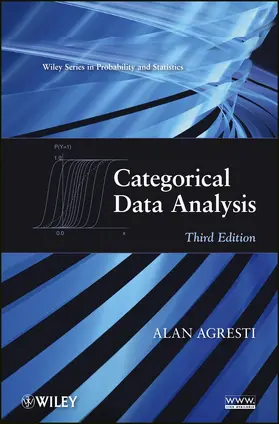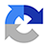# AgrestiCategorical Data Analysis

3. Auflage 2012, 752 Seiten, Gebunden, HC gerader Rücken kaschiert, Format (B × H): 183 mm x 260 mm, Gewicht: 1568 g Reihe: Wiley Series in Probability and Statistics
ISBN: 978-0-470-46363-5
Verlag: Wiley

## AgrestiCategorical Data Analysis

Praise for the Second Edition

"A must-have book for anyone expecting to do research and/or applications in categorical data analysis."
--Statistics in Medicine

"It is a total delight reading this book."
--Pharmaceutical Research

"If you do any analysis of categorical data, this is an essential desktop reference."
--Technometrics

The use of statistical methods for analyzing categorical data has increased dramatically, particularly in the biomedical, social sciences, and financial industries. Responding to new developments, this book offers a comprehensive treatment of the most important methods for categorical data analysis.

Categorical Data Analysis, Third Edition summarizes the latest methods for univariate and correlated multivariate categorical responses. Readers will find a unified generalized linear models approach that connects logistic regression and Poisson and negative binomial loglinear models for discrete data with normal regression for continuous data. This edition also features:

* An emphasis on logistic and probit regression methods for binary, ordinal, and nominal responses for independent observations and for clustered data with marginal models and random effects models
* Two new chapters on alternative methods for binary response data, including smoothing and regularization methods, classification methods such as linear discriminant analysis and classification trees, and cluster analysis
* New sections introducing the Bayesian approach for methods in that chapter
* More than 100 analyses of data sets and over 600 exercises
* Notes at the end of each chapter that provide references to recent research and topics not covered in the text, linked to a bibliography of more than 1,200 sources
* A supplementary website showing how to use R and SAS; for all examples in the text, with information also about SPSS and Stata and with exercise solutions

Categorical Data Analysis, Third Edition is an invaluable tool for statisticians and methodologists, such as biostatisticians and researchers in the social and behavioral sciences, medicine and public health, marketing, education, finance, biological and agricultural sciences, and industrial quality control.

## Weitere Infos & Material

Preface xiii1 Introduction: Distributions and Inference for Categorical Data 11.1 Categorical Response Data, 11.2 Distributions for Categorical Data, 51.3 Statistical Inference for Categorical Data, 81.4 Statistical Inference for Binomial Parameters, 131.5 Statistical Inference for Multinomial Parameters, 171.6 Bayesian Inference for Binomial and Multinomial Parameters, 22Notes, 27Exercises, 282 Describing Contingency Tables 372.1 Probability Structure for Contingency Tables, 372.2 Comparing Two Proportions, 432.3 Conditional Association in Stratified 2 × 2 Tables, 472.4 Measuring Association in I × J Tables, 54Notes, 60Exercises, 603 Inference for Two-Way Contingency Tables 693.1 Confidence Intervals for Association Parameters, 693.2 Testing Independence in Two-way Contingency Tables, 753.3 Following-up Chi-Squared Tests, 803.4 Two-Way Tables with Ordered Classifications, 863.5 Small-Sample Inference for Contingency Tables, 903.6 Bayesian Inference for Two-way Contingency Tables, 963.7 Extensions for Multiway Tables and Nontabulated Responses, 100Notes, 101Exercises, 1034 Introduction to Generalized Linear Models 1134.1 The Generalized Linear Model, 1134.2 Generalized Linear Models for Binary Data, 1174.3 Generalized Linear Models for Counts and Rates, 1224.4 Moments and Likelihood for Generalized Linear Models, 1304.5 Inference and Model Checking for Generalized Linear Models, 1364.6 Fitting Generalized Linear Models, 1434.7 Quasi-Likelihood and Generalized Linear Models, 149Notes, 152Exercises, 1535 Logistic Regression 1635.1 Interpreting Parameters in Logistic Regression, 1635.2 Inference for Logistic Regression, 1695.3 Logistic Models with Categorical Predictors, 1755.4 Multiple Logistic Regression, 1825.5 Fitting Logistic Regression Models, 192Notes, 195Exercises, 1966 Building, Checking, and Applying Logistic Regression Models 2076.1 Strategies in Model Selection, 2076.2 Logistic Regression Diagnostics, 2156.3 Summarizing the Predictive Power of a Model, 2216.4 Mantel-Haenszel and Related Methods for Multiple 2 × 2 Tables, 2256.5 Detecting and Dealing with Infinite Estimates, 2336.6 Sample Size and Power Considerations, 237Notes, 241Exercises, 2437 Alternative Modeling of Binary Response Data 2517.1 Probit and Complementary Log-log Models, 2517.2 Bayesian Inference for Binary Regression, 2577.3 Conditional Logistic Regression, 2657.4 Smoothing: Kernels, Penalized Likelihood, Generalized Additive Models, 2707.5 Issues in Analyzing High-Dimensional Categorical Data, 278Notes, 285Exercises, 2878 Models for Multinomial Responses 2938.1 Nominal Responses: Baseline-Category Logit Models, 2938.2 Ordinal Responses: Cumulative Logit Models, 3018.3 Ordinal Responses: Alternative Models, 3088.4 Testing Conditional Independence in I × J × K Tables, 3148.5 Discrete-Choice Models, 3208.6 Bayesian Modeling of Multinomial Responses, 323Notes, 326Exercises, 3299 Loglinear Models for Contingency Tables 3399.1 Loglinear Models for Two-way Tables, 3399.2 Loglinear Models for Independence and Interaction in Three-way Tables, 3429.3 Inference for Loglinear Models, 3489.4 Loglinear Models for Higher Dimensions, 3509.5 Loglinear--Logistic Model Connection, 3539.6 Loglinear Model Fitting: Likelihood Equations and Asymptotic Distributions, 3569.7 Loglinear Model Fitting: Iterative Methods and Their Application, 364Notes, 368Exercises, 36910 Building and Extending Loglinear Models 37710.1 Conditional Independence Graphs and Collapsibility, 37710.2 Model Selection and Comparison, 38010.3 Residuals for Detecting Cell-Specific Lack of Fit, 38510.4 Modeling Ordinal Associations, 38610.5 Generalized Loglinear and Association Models, Correlation Models, and Correspondence Analysis, 39310.6 Empty Cells and Sparseness in Modeling Contingency Tables, 39810.7 Bayesian Loglinear Modeling, 401Notes, 404Exercises, 40711 Models for Matched Pairs 41311.1 Comparing Dependent Proportions, 41411.2 Conditional Logistic Regression for Binary Matched Pairs, 41811.3 Marginal Models for Square Contingency Tables, 42411.4 Symmetry, Quasi-Symmetry, and Quasi-Independence, 42611.5 Measuring Agreement Between Observers, 43211.6 Bradley-Terry Model for Paired Preferences, 43611.7 Marginal Models and Quasi-Symmetry Models for Matched Sets, 439Notes, 443Exercises, 44512 Clustered Categorical Data: Marginal and Transitional Models 45512.1 Marginal Modeling: Maximum Likelihood Approach, 45612.2 Marginal Modeling: Generalized Estimating Equations (GEEs) Approach, 46212.3 Quasi-Likelihood and Its GEE Multivariate Extension: Details, 46512.4 Transitional Models: Markov Chain and Time Series Models, 473Notes, 478Exercises, 47913 Clustered Categorical Data: Random Effects Models 48913.1 Random Effects Modeling of Clustered Categorical Data, 48913.2 Binary Responses: Logistic-Normal Model, 49413.3 Examples of Random Effects Models for Binary Data, 49813.4 Random Effects Models for Multinomial Data, 51113.5 Multilevel Modeling, 51513.6 GLMM Fitting, Inference, and Prediction, 51913.7 Bayesian Multivariate Categorical Modeling, 523Notes, 525Exercises, 52714 Other Mixture Models for Discrete Data 53514.1 Latent Class Models, 53514.2 Nonparametric Random Effects Models, 54214.3 Beta-Binomial Models, 54814.4 Negative Binomial Regression, 55214.5 Poisson Regression with Random Effects, 555Notes, 557Exercises, 55815 Non-Model-Based Classification and Clustering 56515.1 Classification: Linear Discriminant Analysis, 56515.2 Classification: Tree-Structured Prediction, 57015.3 Cluster Analysis for Categorical Data, 576Notes, 581Exercises, 58216 Large- and Small-Sample Theory for Multinomial Models 58716.1 Delta Method, 58716.2 Asymptotic Distributions of Estimators of Model Parameters and Cell Probabilities, 59216.3 Asymptotic Distributions of Residuals and Goodness-of-fit Statistics, 59416.4 Asymptotic Distributions for Logit/Loglinear Models, 59916.5 Small-Sample Significance Tests for Contingency Tables, 60116.6 Small-Sample Confidence Intervals for Categorical Data, 60316.7 Alternative Estimation Theory for Parametric Models, 610Notes, 615Exercises, 61617 Historical Tour of Categorical Data Analysis 62317.1 Pearson-Yule Association Controversy, 62317.2 R. A. Fisher's Contributions, 62517.3 Logistic Regression, 62717.4 Multiway Contingency Tables and Loglinear Models, 62917.5 Bayesian Methods for Categorical Data, 63317.6 A Look Forward, and Backward, 634Appendix A Statistical Software for Categorical Data Analysis 637Appendix B Chi-Squared Distribution Values 641References 643Author Index 689Example Index 701Subject Index 705Appendix C Software Details for Text Examples (text website)

Agresti, Alan
ALAN AGRESTI is Distinguished Professor Emeritus in the Department of Statistics at the University of Florida. He has presented short courses on categorical data methods in thirty countries. He is the author of five other books, including An Introduction to Categorical Data Analysis, Second Edition and Analysis of Ordinal Categorical Data, Second Edition, both published by Wiley.

## Ihre Fragen, Wünsche oder Anmerkungen

Ihre Nachricht*
Wie möchten Sie kontaktiert werden?
Anrede*
Titel
Vorname*
Nachname*